All Steel Shed Frames, Cabins, Buildings, Connectors, and More... (Phoenix, AZ)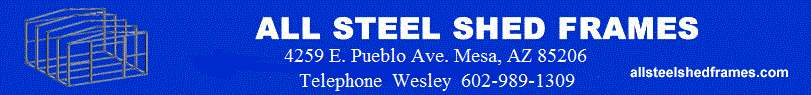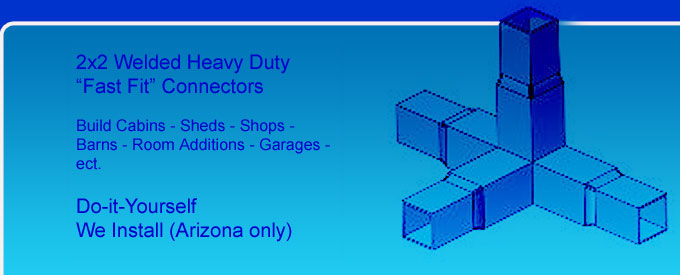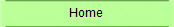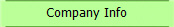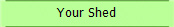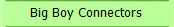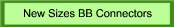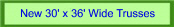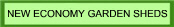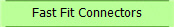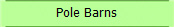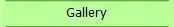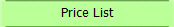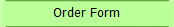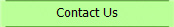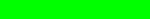>>  "Fast Fit"
Connectors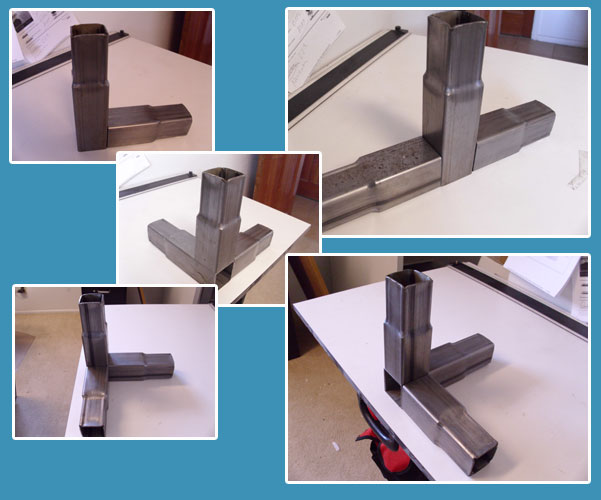ASSF’s New DIY “QUICK BUILD” Portable Steel Frame Building System Allows Homeowner’s To Build
A Quality Storage Shed, Garage Shop or Cabin Easily and in Record Time

All Steel Shed Frames has created the ultimate product for the Do It Yourself builder. It will allow anyone to
build their pet project on a mountain to or in your own back yard.  We offer a unique lifetime steel frame with
“FAST FIT” connectors.  Our connectors are made out of 2 inch sq structural box tubing. All connectors have a
machine induced reduction on the end of each connector allowing it to be inserted into other pieces of steel of
like size. ASSF designed the connectors and framing system to go together as easy as a box full of Lego’s.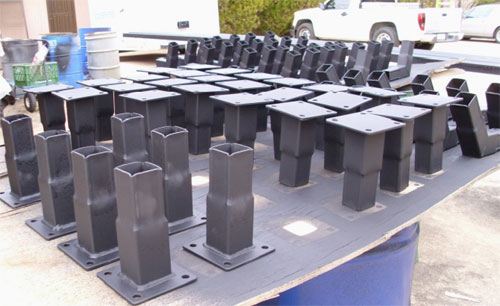ASSF welds the load bearing steel connectors up in the shop to maintain a precision fit and quality control.
These welded connectors have precise welded angles according to our CAD drawings & weighs only a few lbs a
piece. When using our connectors to build a building assembling it’s as easy as inserting one piece of steel into
another.  All Steel Shed Frames 2 ft on center flagship line of portable steel frames that come with “FAST FIT”
connectors are made in 50 sizes and 3 of the most popular roof lines.  Our portable series never needs an
expensive concrete slab under it.

WE SHIP FRAMES NATIONWIDE
FULLY INSTALLED UNITS AVAILABLE

>>  Connectors and Frames Price List

8 FT WIDE STEEL FRAME ONLY

8x6 = \$533      8x8 = \$644      8X10 = \$757      8x12 = \$870

8x14 = \$983       8x16 = \$1096      8X20 = \$1322      8X24 = \$1547

10 FT WIDE STEEL FRAME ONLY

10x10 = \$847      10x12 = \$973      10x14 = \$1099      10x16 = \$1215

10x18 = \$1351      10x20 = \$1477      10x24 = \$1729      10x30 = \$2107

12 FT WIDE STEEL FRAME ONLY

12x12 = \$1051      12x14 = \$1187      12x16 = \$1323      12x18 = \$1459

12x20 = \$1595      12X22 = \$1731      12x24 = \$1864      12x26 = \$2003

12x28 = \$2139      12x30 = \$2275

14 FT WIDE STEEL FRAME ONLY

14x14 = \$1246      14x16 = \$1389      14x18 = \$1532      14x20 = \$1675

14x22 = \$1818      14x24 = \$1961      14X26 = \$2104      14x28 = \$2247

14x30 = \$2400

16 FT WIDE STEEL FRAME ONLY

16X16 = \$1478      16X18 = \$1630      16X20 = \$1782      16X24 = \$2086

16X28 = \$2390      16X32 = \$2694      16X36 = \$2998      16X40 = \$3302

18 FT WIDE STEEL FRAME ONLY

18X18 = \$1793      18X20 = \$1963      18X24 = \$2302      18X28 = \$2643

18X32 = \$2983      18X36 = \$3323      18X40 = \$3663

View the full price list  (PDF Format)

>>  Fully Installed Price List

8 FT WIDE BUILDINGS INSTALLED PRICE

8x6 = \$1465      8x8 = \$1707      8x10 = \$2070      8x12 = \$2378

8x14 = \$2680      8x16 = \$3007      8X20 = \$3673      8X24 = \$4288

10 FT WIDE BUILDINGS INSTALLED PRICE

10x10 = \$2312      10x12 = \$2670      10x14 = \$3052      10x16 = \$3380

10x18 = \$3740     10x20 = \$4104      10x24 = \$4789      10x30 = \$5784

12 FT WIDE BUILDINGS INSTALLED PRICE

12x12 = \$2907      12x14 = \$3300      12x16 = \$3707      12x18 = \$4095

12x20 = \$4496      12x22 = \$4901      12x24 = \$5298      12x26 = \$5717

12x28 = \$6159      12x30 = \$6498

14 FT WIDE BUILDINGS INSTALLED PRICE

14x14 = \$3510      14x16 = \$3979      14x18 = \$4403      14x20 = \$4835

14x22 = \$5320      14x24 = \$5707      14x26 = \$6116      14x28 = \$6549

14x30 = \$6976

16 FT WIDE BUILDINGS INSTALLED PRICE

16X16 = \$4099      16X18 = \$4826      16X20 = \$5355      16X24 = \$6318

16X28 = \$7294      16X32 = \$8270      16X36 = \$9246      16X40 = \$10222

18 FT WIDE BUILDINGS INSTALLED PRICE

18X18 = \$5398      18X20 = \$5943      18X24 = \$7039      18X28 = \$8140

18X32 = \$9232      18X36 = \$10,327      18X40 = \$11,423

View the full price list  (PDF Format)

>>  For More Information

We’d love to answer your questions about our all steel framed portable storage sheds.  For more information
call 602-989-1309 or
send an emailCopyright © 2011   All Steel Shed Frames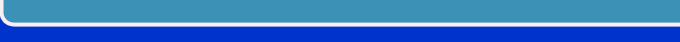Home  |  Company Info  |  Your Shed  |  Big Boy Connectors  |  Fast Fit Connectors  |  Gallery  |  Price List  |  Order Form  |  Contact Us